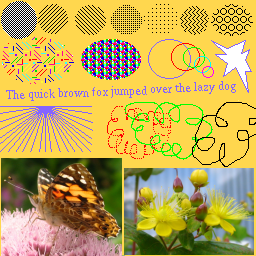The original image.
The following images where shrunk by a factor of 0.55, then zoomed by a factor of 2 to show detail. The weighting curve is different for each image: the top image uses the step curve (source pixel is added to nearest destination pixel), and the bottom uses a linear curve (pixel is weighted according to distance), with the middle images using curves that lie in between step and linear.step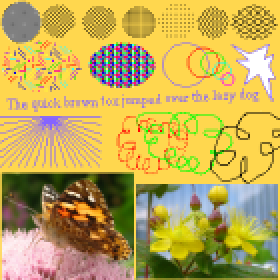steep curve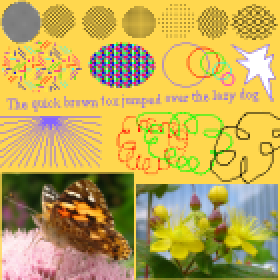less steep curve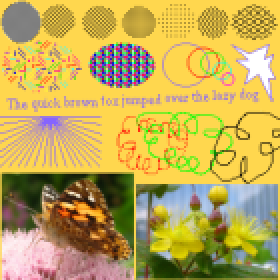even less steep curve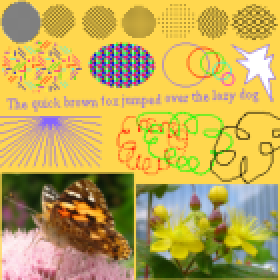sine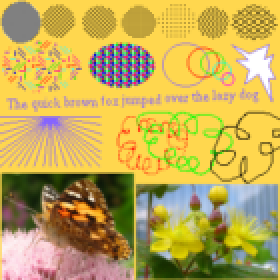linear